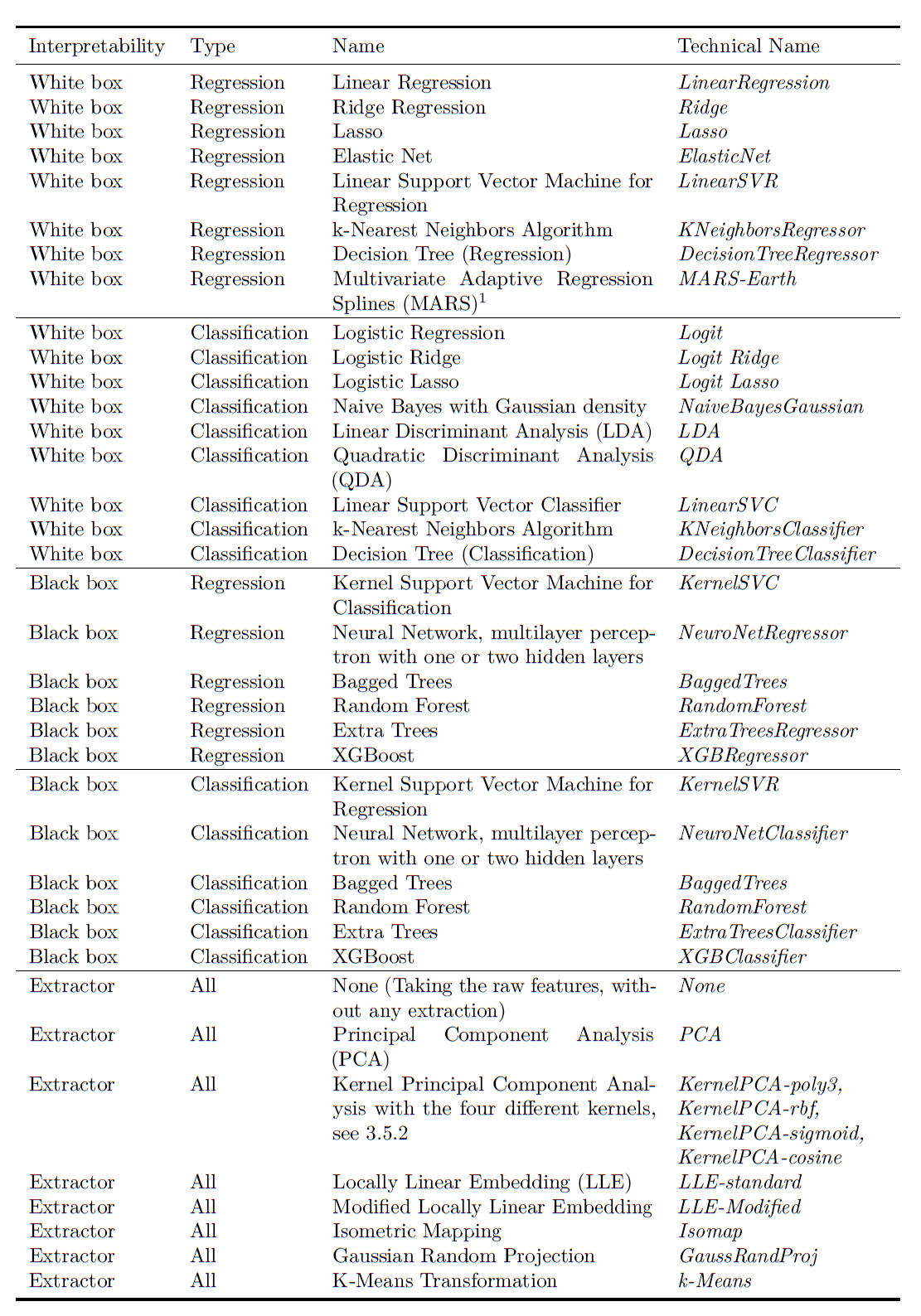# Algorithms

...machine learning is a class of methods for automatically creating models from data. Machine learning algorithms are the engines of machine learning, meaning it is the algorithms that turn a data set into a model. Which kind of algorithm works best (supervised, unsupervised, classification, Regression, etc.) depends on the kind of problem you’re solving, the computing resources available, and the nature of the data. Ordinary programming algorithms tell the computer what to do in a straightforward way. For example, sorting algorithms turn unordered data into data ordered by some criteria, often the numeric or alphabetical order of one or more fields in the data. Machine learning algorithms explained | Martin Heller - InfoWorld

• Linear Regression algorithms fit a straight line, or another function that is linear in its parameters such as a polynomial, to numeric data, typically by performing matrix inversions to minimize the squared error between the line and the data. Squared error is used as the metric because you don’t care whether the Regression line is above or below the data points; you only care about the distance between the line and the points.
• Nonlinear Regression algorithms, which fit curves that are not linear in their parameters to data, are a little more complicated, because, unlike linear Regression problems, they can’t be solved with a deterministic method. Instead, the nonlinear Regression algorithms implement some kind of iterative minimization process, often some variation on the method of steepest descent.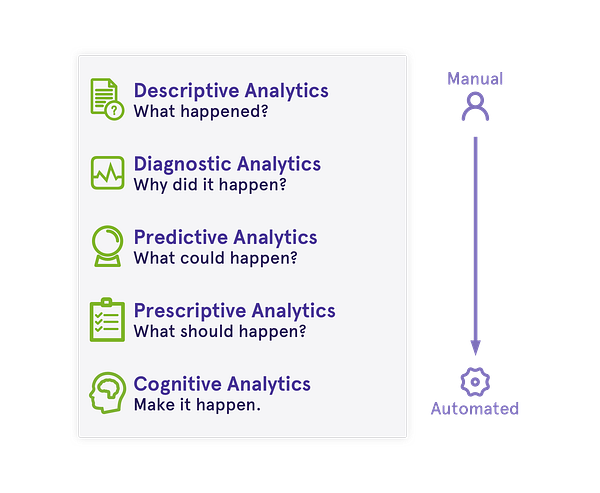Predictive and Preventive | Vinay Mehendiratta - KDnuggets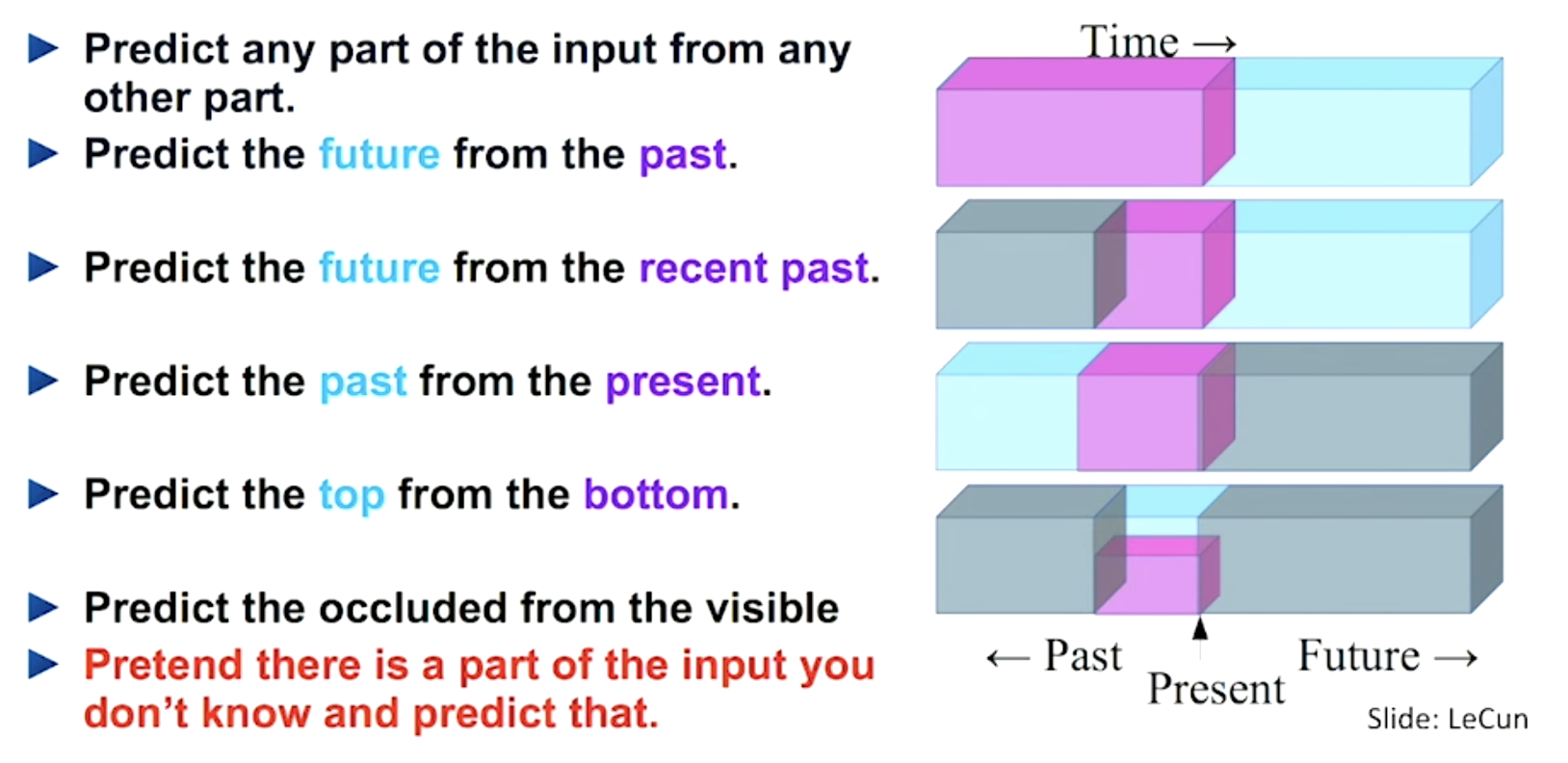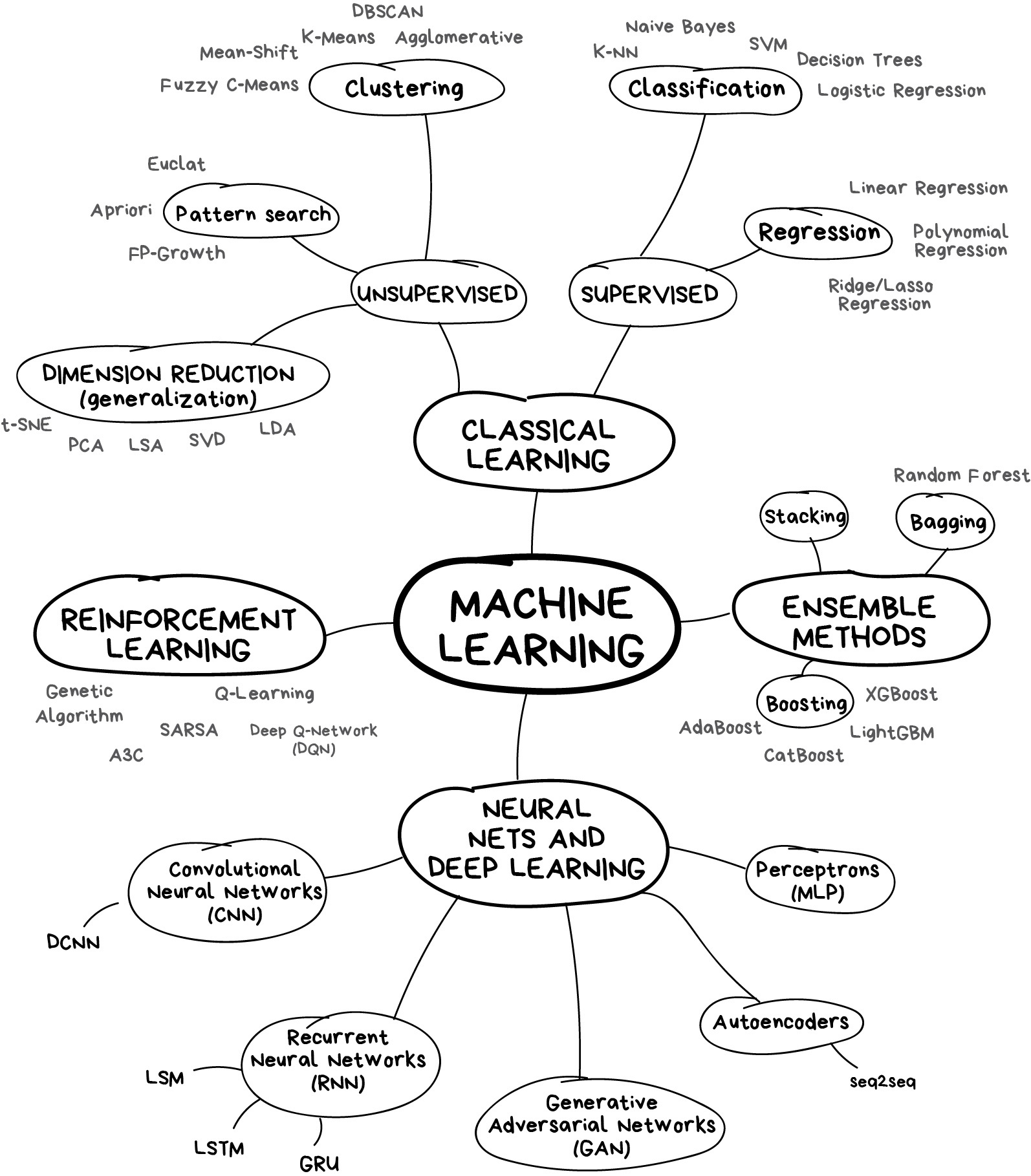## Neural Networks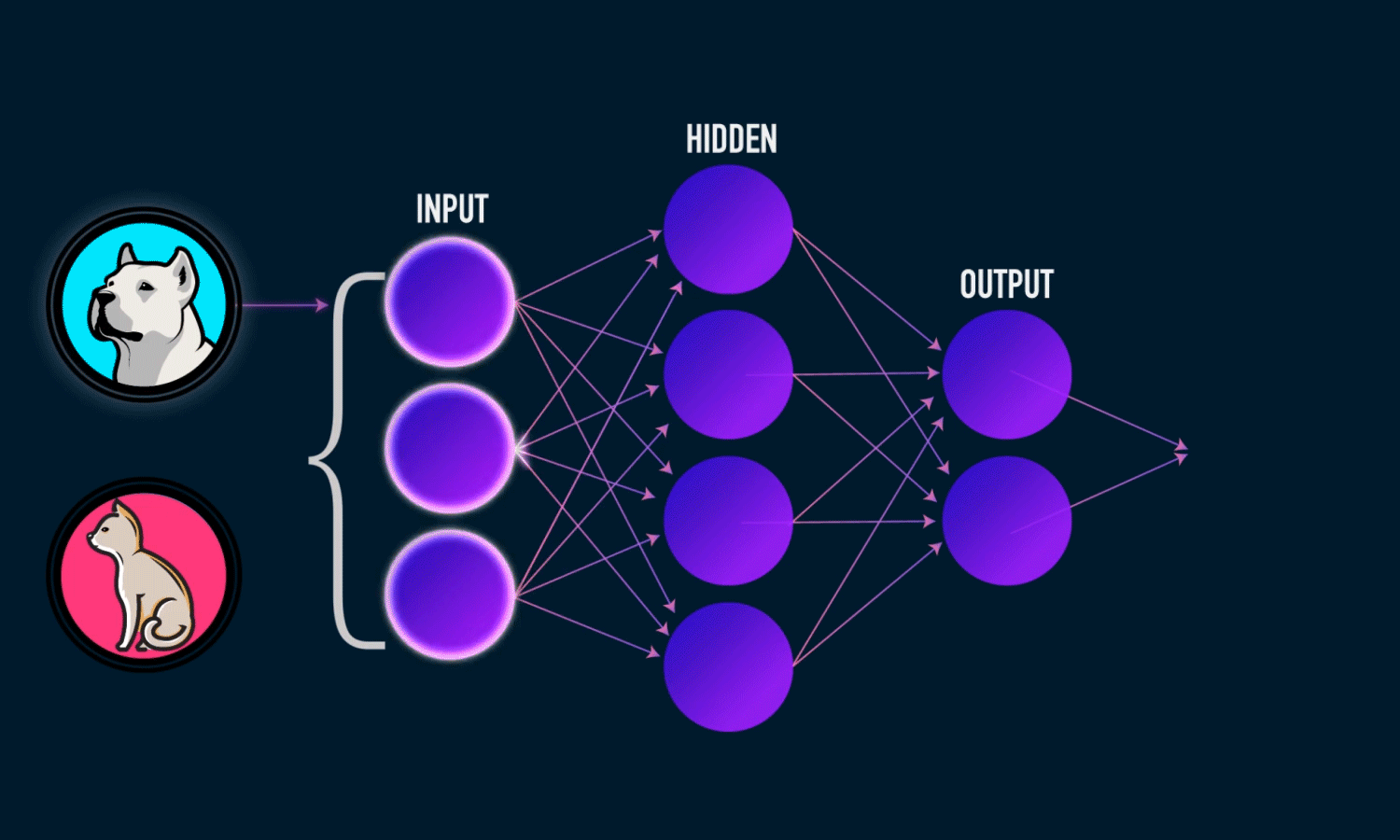## How often do data scientist jobs require data scientists to develop machine learning models from scratch?

Models? All the time. But you probably meant machine learning algorithms. In that case, the answer is almost never.

It is very rare that entirely new algorithms show up at all. How many significant breakthroughs did we see in this decade? Generative Adversarial Network (GAN) is one that comes to mind. The attention mechanism in neural machine translation is another one (as suggested by Sathvik Udupa). Both were innovations that came from academia.

In industry, DeepMind has come up with some interesting work, like Deep Q-learning, Monte Carlo Tree Search and Neural Turing Machines, but these were mostly based on previous research.

Very few data scientists are working on new algorithms. This is something that happens almost exclusively in industrial R&D labs. Not many companies in the world can afford the luxury of working on something as fundamental as new machine learning algorithms. It’s a big investment for a tiny chance that something useful materializes.

Data scientists solve real-world problems, and are subjected to time and cost constraints. There’s also something called the “no free lunch” theorem. Basically, it means that no optimization algorithm can outperform others when averaged over all problems. Although a very specific algorithm could potentially outperform standard algorithms on a very specific problem, it’s just not worth the time and effort to invest in it. Even if the team by some miracle manages to outperform standard algorithms, it’s almost certainly going to be a small incremental gain and not revolutionary. Håkon Hapnes Strand - LinkedIn